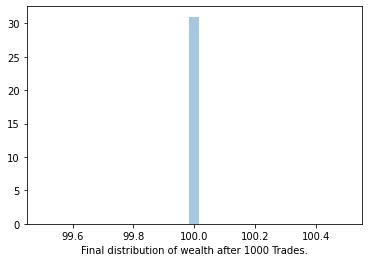None

Here I'm trying to simulate a trade game between N people, everybody holds M unit of money. After doing P transactions we want to know what's the final wealth distribution of each people. I'm doing this to study the realworld wealth disparity using a simple trade simulation.

• N = 1000 People
• M = 100 unit of money
• P = 1000, 10000, 1000000 number of transactions

1. Randomly pick two people from 1000.
2. Toss a coin and if it returns head, first person gets 1 unit of money from second person or vice versa.

Want to play with this notebook - Try out here

# !pip install seaborn numpy scipy

from collections import Counter
import seaborn as sns
import numpy as np
import scipy

start_state = np.ones(1000) * 100

def start_trade(num_trades=1000, num_people=1000, drop_zero=True, plot=True):
# Starts with 100 unit of Money equally allocated to 1000 people.
start_state = np.ones(num_people) * 100
person_indexes = range(0, num_people)
# Using PRNG to get samples from uniform distribution, it's not ideal but
# good enough for most of the times.
get_person_id = lambda: np.random.choice(person_indexes)
toss = lambda: np.random.choice(["H", "T"])

# picking two people randomly for trade.
# p1 = get_person_id()
# p2 = get_person_id()
p1 = get_random_person_id(start_state, drop_zero)
p2 = get_random_person_id(start_state, drop_zero)

# Toss
t = toss()

#If it's head, first person gets the money or second person.
if t == "H":
start_state[p1] += 1
start_state[p2] -= 1
else:
start_state[p1] -= 1
start_state[p2] += 1
return start_state

def get_random_person_id(state: np.array, drop_zero=False):
"""
Randomly pick a person from list of persons.

Array index is person_id.
"""
if drop_zero:
state = np.argwhere(state > 0).squeeze()
else:
state = np.argwhere(state).squeeze()
return np.random.choice(state)

''' plot the final state of the trade'''
plot = sns.distplot(
nums,
fig = plot.get_figure()
fig.savefig(figname)


# How to interpret the distribution graph¶

Here we are ploting the outcome that traders get from multiple trades between N number of people and then done a distribution plot. That means it generats a Probabilistics Distribution plot over the outcomes here.

We can call the outcome (a random variable) here as the outcome or final wealth of a person after doing N random trades with M number of people.

The distribution plot has a estimated line line plot, area under that will be always 1. Now you can interpret this in terms of percentage / probability.

Assume you have played the #4 trade mentioend below, ie; Doing 1 Million transactions, here you can clearly see a bell curve, that means after the game ~50% of time you will gain money, and ~50% of time you will lose money. But at every stage the loss / gain can be different impact to that person, because loss is more impactful if a person loss 1 rupee from 50 compared to a loss of 1 rupee from total of 150. This shows that even if the game is fair the loss isn't fair that shows why at the end of the game we are seeing a distribution, and faviouring those who has more accumulation.

Another key point is most of the plots average return (Expected value) is less than 100, that means you are most likely going to lose money.

NOTE: ~50% means approximately 50%

# 1. Trade between 1000 people¶

Multiple experiments are ran with different number of trades. That helps to see how the final wealth distribution will look like if all of the people do the trade based on coin toss.

## 1.1. Do Thousand Transactions¶

num_trades = 1000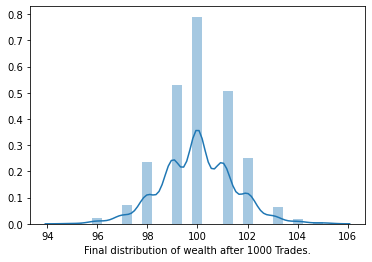## 1.2. Do Ten Thousand Transactions¶

num_trades = 10000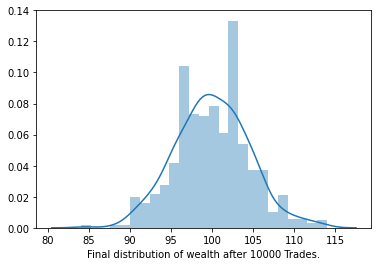## 1.3. Do One Lac Transactions¶

num_trades = 100000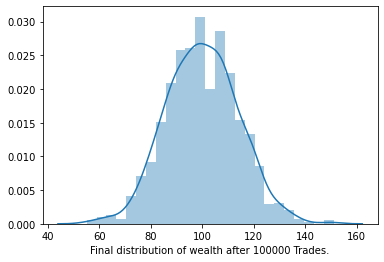## 1.4. Do One Million Transactions¶

num_trades = 1000000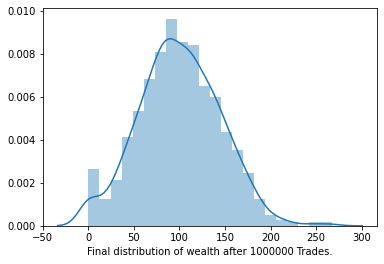## 1.5 Quick check on the PDF¶

# Pick out the Probability Density Function and see what's the probability of getting
# 100 when you play this game ?
hist = np.histogram(final_state, bins=20)
hist_dist = scipy.stats.rv_histogram(hist)

hist_dist.pdf(100.0) * 100  # ie; not even 1% chance you get 100 afte this trade.

0.8070175438596492

# 2. Trade between 2 people¶

Starts with 100 unit of cash. If we play this N number of times this also converges to bell curve (Normal distribution).

num_trades = 20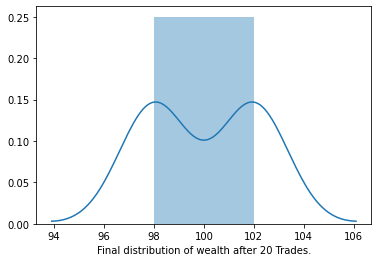num_trades = 1000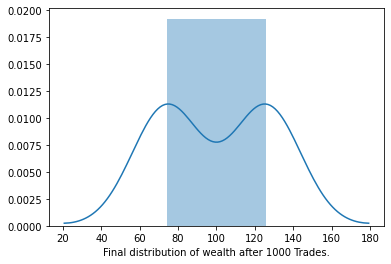num_trades = 100000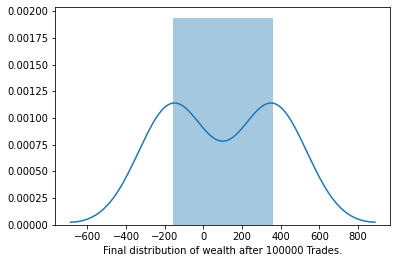num_trades = 1000000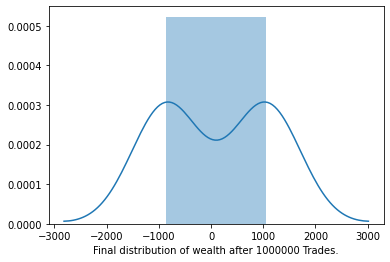# 3. Trade for 1% of wealth¶

Previous case each traders lose or gain 1 unit of cash, which is an absolute value. Let's make it a 1% of his wealth will be lost when he lose the trade. Here We are making it relative to check that the final wealth distribution uniform or not.

def start_trade_v2(num_trades=1000, num_people=1000, plot=True):
# Starts with 100 unit of Money equally allocated to 1000 people.
start_state = np.ones(num_people) * 100
person_indexes = range(0, num_people)
# Using PRNG to get samples from uniform distribution, it's not ideal but
# good enough for most of the times.
get_person_id = lambda: np.random.choice(person_indexes)
toss = lambda: np.random.choice(["H", "T"])

# picking two people randomly for trade.
p1 = get_person_id()
p2 = get_person_id()

# Toss
t = toss()

#If it's head, first person gets the money or second person.
w1 = start_state[p1]
w2 = start_state[p2]
if t == "H":
# Penalty is 1% of the w2's wealth
start_state[p1] += (w2 / 100)
start_state[p2] -= (w2 / 100)
else:
start_state[p1] -= (w1 / 100)
start_state[p2] += (w1 / 100)
return start_state

num_trades = 1000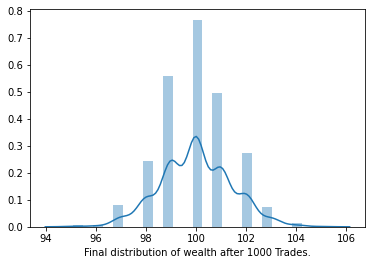# 3. No loss if below particular level¶

Let's assume the scenario that if the wealth of a person is below 100, then he don't have to give penalty. If a person has wealth equal or more than 100 then he has to give penalty of 1 if he fails. Let's see how this will come.

def start_trade_v3(num_trades=1000, num_people=1000, plot=True):
# Starts with 100 unit of Money equally allocated to 1000 people.
start_state = np.ones(num_people) * 100
person_indexes = range(0, num_people)
# Using PRNG to get samples from uniform distribution, it's not ideal but
# good enough for most of the times.
get_person_id = lambda: np.random.choice(person_indexes)
toss = lambda: np.random.choice(["H", "T"])

# picking two people randomly for trade.
p1 = get_person_id()
p2 = get_person_id()

# Toss
t = toss()

#If it's head, first person gets the money or second person.
w1 = start_state[p1]
w2 = start_state[p2]
w1p = 0
w2p = 0
if w1 >= 100:
w1p = 1
if w2 >= 100:
w2p = 1

if t == "H":
# Penalty is 1% of the w2's wealth
start_state[p1] += w2p
start_state[p2] -= w2p
else:
start_state[p1] -= w1p
start_state[p2] += w1p
return start_state

num_trades = 100000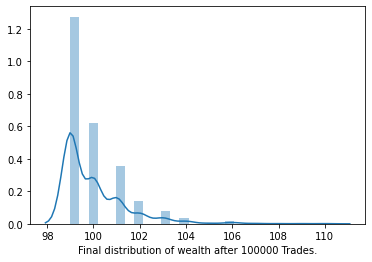Here also Norml or close to power law distribution. Again more people lose money it seems.

# 4. You loss all your gains if you fail¶

Another attempt to get equal / uniform distribution after the N trades. In this case the rule is, who ever losing the toss has to lose whatever trader earned extra since beginning.

This setting giving the uniform distribution for everybody has 100 each at the end of the trade also.

def start_trade_v4(num_trades=1000, num_people=1000, plot=True):
# Starts with 100 unit of Money equally allocated to 1000 people.
start_state = np.ones(num_people) * 100
person_indexes = range(0, num_people)
# Using PRNG to get samples from uniform distribution, it's not ideal but
# good enough for most of the times.
get_person_id = lambda: np.random.choice(person_indexes)
toss = lambda: np.random.choice(["H", "T"])

# picking two people randomly for trade.
p1 = get_person_id()
p2 = get_person_id()

# Toss
t = toss()

#If it's head, first person gets the money or second person.
w1 = start_state[p1]
w2 = start_state[p2]
w1p = 0
w2p = 0
if w1 >= 100:
w1p = w1 - 100
if w2 >= 100:
w2p = w2 - 100

if t == "H":
# Penalty is 1% of the w2's wealth
start_state[p1] += w2p
start_state[p2] -= w2p
else:
start_state[p1] -= w1p
start_state[p2] += w1p
return start_state

num_trades = 1000

/Users/haridas/ENV3/lib/python3.7/site-packages/seaborn/distributions.py:283: UserWarning: Data must have variance to compute a kernel density estimate.